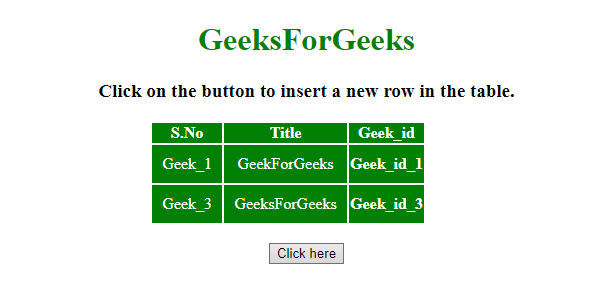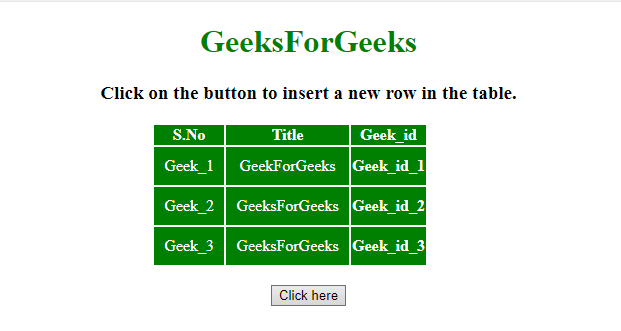Related Articles

# How to insert new row at a certain index in a table in jQuery ?

• Last Updated : 28 Jun, 2019

Given an HTML document containing a table. The task is to insert a new row in that table at a certain index using JQuery.

Approach:

Hey geek! The constant emerging technologies in the world of web development always keeps the excitement for this subject through the roof. But before you tackle the big projects, we suggest you start by learning the basics. Kickstart your web development journey by learning JS concepts with our JavaScript Course. Now at it's lowest price ever!

• Store the table column value <td> element into the variable.
• Then use eq() and after() method to insert the row in a table.

Example 1: In this example, the row is inserted at index 1 (hardcoded).

 ` ``<``html``> ``    ``<``head``> ``        ``<``title``> ``            ``Insert new row at a certain index``            ``in a table using jQuery``        `` ``         ` `        ``<``script` `src` `= ``"https://ajax.googleapis.com/ajax/libs/jquery/3.4.0/jquery.min.js"``>``        ````         ` `        ``<``style``>``            ``#myCol {``                ``background:green;``            ``}``            ``table {``                ``color:white;``            ``}``            ``#Geek_p {``                ``color:green;``                ``font-size:30px;``            ``}``            ``td {``                ``padding:10px;``            ``}``        ````    ````     ` `    ``<``body``> ``        ``<``center``>    ``        ``<``h1` `style` `= ``"color:green;"` `> ``            ``GeeksForGeeks ``        `` ``         ` `        ``<``strong``>``            ``Click on the button to insert``            ``a new row in the table``        ````         ` `        ``<``br``><``br``>``         ` `        ``<``table``>``            ``<``colgroup``>``                ``<``col` `id``=``"myCol"``                    ``span``=``"2"``>``                ``<``col` `style``=``"background-color:green"``>``            ````             ` `            ``<``tr``>``                ``<``th``>S.No``                ``<``th``>Title``                ``<``th``>Geek_id``            ````            ``<``tr` `id` `= ``"row1"``>``                ``<``td``>Geek_1``                ``<``td``>GeekForGeeks``                ``<``th``>Geek_id_1``            ````            ``<``tr``>``                ``<``td``>Geek_3``                ``<``td``>GeeksForGeeks``                ``<``th``>Geek_id_3``            ````        ````        ``<``br``>``         ` `        ``<``button` `onclick` `= ``"Geeks()"``> ``            ``Click here``        `` ``         ` `        ````        ``<``script``> ``            ``function Geeks() {``                ``var html = ``                ``"<``td``>Geek_2<``td``>GeeksForGeeks<``th``>Geek_id_2";``                 ` `                ``\$('table > tbody > tr').eq(1).after(html);``            ``}``        `` ``        ````    `` ``                    `

Output:

• Before clicking on the button:• After clicking on the button:Example 2: In this example, the row is inserted at index provided by the user.

 ` ``<``html``> ``    ``<``head``> ``        ``<``title``> ``            ``Insert new row at a certain index``            ``in a table using jQuery``        `` ``         ` `        ``<``script` `src` `= ``"https://ajax.googleapis.com/ajax/libs/jquery/3.4.0/jquery.min.js"``>``        ````         ` `        ``<``style``>``            ``#myCol {``                ``background:green;``            ``}``            ``table {``                ``color:white;``            ``}``            ``#Geek_p {``                ``color:green;``                ``font-size:30px;``            ``}``            ``td {``                ``padding:10px;``            ``}``        ````    ````     ` `    ``<``body``> ``        ``<``center``>    ``        ``<``h1` `style` `= ``"color:green;"` `> ``            ``GeeksForGeeks ``        `` ``         ` `        ``<``strong``>``            ``Click on the button to insert``            ``a new row in the table``        ````         ` `        ``<``br``><``br``>``         ` `        ``<``table``>``            ``<``colgroup``>``                ``<``col` `id``=``"myCol"``                    ``span``=``"2"``>``                ``<``col` `style``=``"background-color:green"``>``            ````             ` `            ``<``tr``>``                ``<``th``>S.No``                ``<``th``>Title``                ``<``th``>Geek_id``            ````            ``<``tr` `id` `= ``"row1"``>``                ``<``td``>Geek_1``                ``<``td``>GeekForGeeks``                ``<``th``>Geek_id_1``            ````            ``<``tr``>``                ``<``td``>Geek_3``                ``<``td``>GeeksForGeeks``                ``<``th``>Geek_id_3``            ````        ````        ``<``br``>``         ` `        ``<``button` `onclick` `= ``"Geeks()"``> ``            ``Click here``        `` ``         ` `        ````        ``<``script``> ``            ``function Geeks() {``                ``var i = 2;``                ``var html = ``                ``"<``td``>Geek_2<``td``>GeeksForGeeks<``th``>Geek_id_2";``                 ` `                ``\$('table > tbody > tr').eq(i - 1).after(html);``            ``}``        `` ``        ````    `` ``                    `

Output:

• Before clicking on the button:• After clicking on the button:My Personal Notes arrow_drop_up